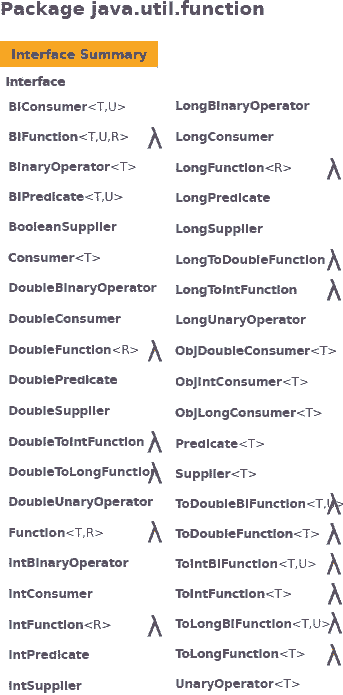# 如何充分利用Java Function接口？

19-02-21 banq

Java Function接口非常简单。它将单个Java对象作为参数，并在方法结束时返回单个Java对象。您可以想出的任何方法都会获取一个对象并返回一个满足Java函数契约的对象。

 ```java.util.function.Function Java Interface Function Parameter Types: T - the input given to the function R - the result running the function Popular Subinterface of Function: UnaryOperator ```

 ```R apply(T t) ```

 ```class FunctionClass implements Function {   public String apply(Integer t) {     return Integer.toString(t*t);   } } ```

 ```Function functionClass = new FunctionClass(); System.out.println(functionClass.apply(2)); ```

Java Function接口示例

 ```Function innerClass = new Function(){   public String apply(Integer t) {     return Integer.toString(t*t);   } }; System.out.println(innerClass.apply(3)); ```

Java的Function和lambda表达式示例

 ```Function verboseLambda = (Integer x)-> { return Integer.toString(x*x); }; System.out.println(verboseLambda.apply(5)); ```

 ```Function conciseLambda = (Integer x)-> { return Integer.toString(x*x); }; System.out.println(conciseLambda.apply(5)); ```